﻿ ProjectAsync(IEnumerable<Graphic>,SpatialReference,DatumTransform,Boolean,Object) Method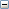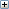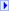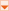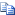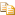Collapse All Expand AllLanguage Filter: All Language Filter: Multiple Language Filter: Visual Basic (Declaration) Language Filter: C#
 ArcGIS API for Silverlight - Library Reference ProjectAsync(IEnumerable,SpatialReference,DatumTransform,Boolean,Object) Method See Also  Example Send comments on this topic
 ESRI.ArcGIS.Client.Tasks Namespace > GeometryService Class > ProjectAsync Method : ProjectAsync(IEnumerable,SpatialReference,DatumTransform,Boolean,Object) Method

graphics
The graphics containing the geometry to project.
outSpatialReference
The SpatialReference to project to.
datumTransform
The datum transformation object. Support for DatumTransfom works with ArcGIS Server 10.1+
transformForward
If DatumTransform is provided transformFoward value will indicate to project datum forward if true or backwards if false. Support for DatumTransfom works with ArcGIS Server 10.1+
userToken
A user-defined object that is passed to the method invoked.
Projects a set of Graphics into a new SpatialReference including a DatumTransform. Raises the ProjectCompleted event on success, or the TaskBase.Failed event on failure; requires ArcGIS Server 10.1 or higher.

#Syntax

Visual Basic (Declaration)
```Public Overloads Sub ProjectAsync( _
ByVal graphics As IEnumerable(Of Graphic), _
ByVal outSpatialReference As SpatialReference, _
ByVal datumTransform As DatumTransform, _
Optional ByVal transformForward As Boolean, _
Optional ByVal userToken As Object _
) ```
C#
```public void ProjectAsync(
IEnumerable<Graphic> graphics,
SpatialReference outSpatialReference,
DatumTransform datumTransform,
bool transformForward,
object userToken
)```

#Remarks

The SpatialReference is a combination of an ellipsoid, datum, and a coordinate system used to display geographic data of the three dimensional Earth on a two dimensional surface (such as a piece of paper of computer monitor). A good article for describing how a spatial reference works can be found here.

The ProjectAsync Method can be used to project Graphics in the Projected Coordinate Systems and Geographic Coordinate Systems. The Well-known ID (aka. WKID) values are listed in these hyperlinked Coordinate System documents and serve as integer values when creating a new SpatialReference object using the constructor.

Excellent background information about map projects can be found in the ArcGIS Desktop Resource Center under the nodes of Professional Library | Guide books | Map projections. A good starting page is the "What are map projects?" web document.

This version of the ProjectAsync Method also requires performing a DatumTransform as part of generating new Graphics in a different projection. While a spheroid approximates the shape of the earth, a datum defines the position of the spheroid relative to the center of the earth. A datum provides a frame of reference for measuring locations on the surface of the earth. It defines the origin and orientation of latitude and longitude lines. See the ArcGIS Resource Center web documents Spheroids and spheres and Datums for more theoretical documentation.

Constructing the DatumTransform object (which is the 3rd input parameter of this ProjectAsync Method) requires knowing the Well-known ID (aka. WKID) for Datum Transformations. A list of all of these WKID Datum Transformations values can be found by scrolling down to the bottom of the Table of Contents and clicking on the Datum Transformation list hyperlink in the ArcGIS Server REST API for your ArcGIS Server installation. See the following screen shot: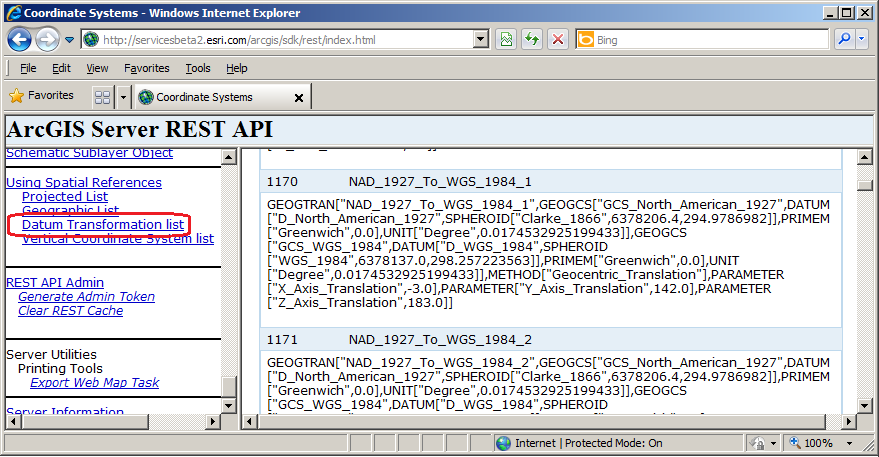As of the publishing of this API document, the list of WKID for Data Transformations can be found here on a public ArcGIS 10.1 test server.

For example: using the DatumTransform object with the WKID of 1170 (Name: NAD_1927_To_WGS_1984_1) means that a datum transformation can be performed between the Clarke 1866 spheroid and World Geodetic System (WGS) 1984 spheroid.

The optional 4th parameter (transformForward) of this version of the ProjectAsync Method takes a Boolean value to indicate which direction of the Datum Transformation will occur. In the case of the WKID of 1170, a transformForward = true means the transformation will be from the Clarke 1866 spheroid to the World Geodetic System (WGS) 1984 spheroid. If the transformForward = false, then the transformation will be from the World Geodetic System (WGS) 1984 spheroid to the Clarke 1866 spheroid. By default, if the optional 4th parameter of transformForward is not specified, then a ProjectAsync Method automatically infers that the transformForward = true for the specified DatumTransform WKID.

It is required that the first parameter (IEnumerable<Graphic>)of this version of ProjectAsync have a different SpatialReference than the second parameter in order to have valid DatumTansform return values in the GeometryService.ProjectCompleted Event. It is not possible to have Graphics in the first parameter have the same SpatialReference as the second parameter and just apply the DatumTransform; that is not how the service works. For example: if the list of Graphics were based upon the SpatialReference of WKID of 4326, you could not use the SpatialReference of WKID 4326 as the second parameter of this version of the ProjectAsync Method and get back valid results in the GeometryService.ProjectCompleted Event for a DatumTransform.

This version of ProjectAsync requires ArcGIS Server 10.1 or higher in order to have valid DatumTransforms Graphics returned in the GeometryService.ProjectCompleted Event. If you attempt to use this version of ProjectAsync on a GeometryService that is based on a version of ArcGIS Server earlier than 10.1, you will obtain Graphics returned in the GeometryService.ProjectCompleted Event but the Graphics will not have the DatumTransform changes applied.

#### Parameters

graphics
The graphics containing the geometry to project.
outSpatialReference
The SpatialReference to project to.
datumTransform
The datum transformation object. Support for DatumTransfom works with ArcGIS Server 10.1+
transformForward
If DatumTransform is provided transformFoward value will indicate to project datum forward if true or backwards if false. Support for DatumTransfom works with ArcGIS Server 10.1+
userToken
A user-defined object that is passed to the method invoked.

#Example

How to use:

Click on the map to display various ProjectAsync Graphics. The location where the user clicks does not use a Datum Transform (this will display as a Red Circle). Depending on which radio button is chosen (Forward or Backward) will also cause add another Graphic to appear using the ProjectAsync Method but with a Datum Transform applied. The Cyan Square represents a Forward Datum Transform and the Black Square represents a Backward Datum Transform.

The XAML code in this example is used in conjunction with the code-behind (C# or VB.NET) to demonstrate the functionality.

The following screen shot corresponds to the code example in this page.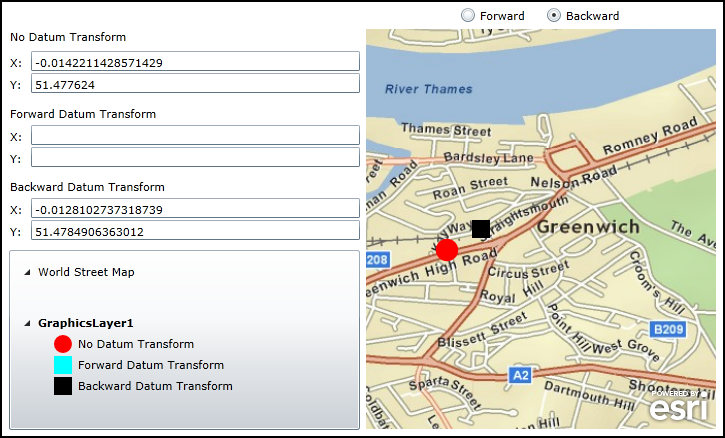XAMLCopy Code
```<Grid x:Name="LayoutRoot">

<!--
Create a set of Symbols that will be used to render the Graphics. Use a UniqueValueRenderer to automatically
seperate out the Graphics into groups based upon the Graphic.Attribute.
-->
<Grid.Resources>

<!-- There will be three unique Symbols. -->
<esri:SimpleMarkerSymbol x:Key="DefaultMarkerSymbol" Color="Red" Size="18" Style="Circle" />
<esri:SimpleMarkerSymbol x:Key="ForwardTransform" Color="Cyan" Size="18" Style="Square" />
<esri:SimpleMarkerSymbol x:Key="BackwardTransform" Color="Black" Size="18" Style="Square" />

<!--
Bind the symbols of the UniqueValueRenderer to each of the three unique Symbols. The basis of the
UniqueValueRenderer categorizations comes from the Attribute Field called 'Direction'. Each
UniqueValueInfo catergory matches what is in the 'Direction' field. The DefaultSymbol acts as
a catch-all if the Value for a UniqueValueInfo category is not matched.
-->
<esri:UniqueValueRenderer x:Key="renderer" DefaultSymbol="{StaticResource DefaultMarkerSymbol}"
DefaultLabel="No Datum Transform" Field="Direction">
<esri:UniqueValueRenderer.Infos>
<esri:UniqueValueInfo Label="Forward Datum Transform" Symbol="{StaticResource ForwardTransform}" Value="Forward"/>
<esri:UniqueValueInfo Label="Backward Datum Transform" Symbol="{StaticResource BackwardTransform}" Value="Backward"/>
</esri:UniqueValueRenderer.Infos>
</esri:UniqueValueRenderer>
</Grid.Resources>

<!-- Display X and Y information for the location where the user clicked on the map. -->
<sdk:Label Height="20" HorizontalAlignment="Left" Margin="13,217,0,0" Name="Label_NoDatumTransform_X"
VerticalAlignment="Top" Width="15" Content="X:"/>
<TextBox Height="20" HorizontalAlignment="Left" Margin="34,213,0,0" Name="TextBox_NoDatumTransform_X"
VerticalAlignment="Top" Width="329" />
<sdk:Label Height="20" HorizontalAlignment="Left" Margin="13,239,0,0" Name="Label_NoDatumTransform_Y"
VerticalAlignment="Top" Width="15" Content="Y:"/>
<TextBox Height="20" HorizontalAlignment="Left" Margin="34,235,0,0" Name="TextBox_NoDatumTransform_Y"
VerticalAlignment="Top" Width="329" />
<sdk:Label Height="20" HorizontalAlignment="Left" Margin="13,191,0,0" Name="Label_NoDatumTransform"
VerticalAlignment="Top" Width="350" Content="No Datum Transform"/>

<!-- Display X and Y information for the Datum Transform Forward. -->
<sdk:Label Content="X:" Height="20" HorizontalAlignment="Left" Margin="13,291,0,0" Name="Label_ForwardDatumTransform_X"
VerticalAlignment="Top" Width="15" />
<TextBox Height="20" HorizontalAlignment="Left" Margin="34,287,0,0" Name="TextBox_ForwardDatumTransform_X"
VerticalAlignment="Top" Width="329" />
<sdk:Label Content="Y:" Height="20" HorizontalAlignment="Left" Margin="13,313,0,0" Name="Label_ForwardDatumTransform_Y"
VerticalAlignment="Top" Width="15" />
<TextBox Height="20" HorizontalAlignment="Left" Margin="34,309,0,0" Name="TextBox_ForwardDatumTransform_Y"
VerticalAlignment="Top" Width="329" />
<sdk:Label Content="Forward Datum Transform" Height="20" HorizontalAlignment="Left" Margin="13,268,0,0"
Name="Label_ForwardDatumTransform" VerticalAlignment="Top" Width="350" />

<!-- Display X and Y information for the Datum Transform Backward. -->
<sdk:Label Content="X:" Height="20" HorizontalAlignment="Left" Margin="13,364,0,0" Name="Label_BackwardDatumTransform_X"
VerticalAlignment="Top" Width="15" />
<TextBox Height="20" HorizontalAlignment="Left" Margin="34,360,0,0" Name="TextBox_BackwardDatumTransform_X"
VerticalAlignment="Top" Width="329" />
<sdk:Label Content="Y:" Height="20" HorizontalAlignment="Left" Margin="13,386,0,0" Name="Label_BackwardDatumTransform_Y"
VerticalAlignment="Top" Width="15" />
<TextBox Height="20" HorizontalAlignment="Left" Margin="34,382,0,0" Name="TextBox_BackwardDatumTransform_Y"
VerticalAlignment="Top" Width="329" />
<sdk:Label Content="Backward Datum Transform" Height="20" HorizontalAlignment="Left" Margin="13,341,0,0" Name="Label_BackwardDatumTransform"
VerticalAlignment="Top" Width="350" />

<!-- Allow the user options to create a Forward or Backward Datum Transform. -->
<RadioButton Content="Forward" Height="16" HorizontalAlignment="Left" Margin="463,169,0,0" Name="rb_Forward"
VerticalAlignment="Top" IsChecked="True"/>
<RadioButton Content="Backward" Height="16" HorizontalAlignment="Left" Margin="549,169,0,0" Name="rb_Backward"
VerticalAlignment="Top" />

<!--
Add a Map Control zoomed into Greenwich, England. The ArcGISTiledMapServiceLayer that is added
dictates that the Spatial Reference of the Map Control (which is WKID 4326). Add a GraphicLayer
that will house where the user clicks and the various Datum Transforms. The Symbology of the
GraphicLayer is bound the StaticResources define above.
-->
<esri:Map Background="White" HorizontalAlignment="Left" Margin="369,191,0,0"
Name="Map1" VerticalAlignment="Top" WrapAround="True" Height="400" Width="350"
Extent="-0.0176,51.476,-0.003,51.481">
<esri:Map.Layers>
<esri:LayerCollection>
<esri:ArcGISTiledMapServiceLayer
Url="http://services.arcgisonline.com/ArcGIS/rest/services/ESRI_StreetMap_World_2D/MapServer" />
<esri:GraphicsLayer ID="GraphicsLayer1" Renderer="{StaticResource renderer}" />
</esri:LayerCollection>
</esri:Map.Layers>
</esri:Map>

<!-- Add a Legend bound to the Map control to display the Graphics added. -->
<esri:Legend HorizontalAlignment="Left" Margin="12,412,0,0" Name="Legend1" VerticalAlignment="Top" Width="351"
Height="180" Map="{Binding ElementName=Map1}" />

<!-- Provide the instructions on how to use the sample code. -->
<TextBlock Height="104" HorizontalAlignment="Left" Name="TextBlock1" VerticalAlignment="Top" Width="788"
TextWrapping="Wrap" Text="Click on the map to display various ProjectAsync Graphics. The location
where the user clicks does not use a Datum Transform (this will display as a Red Circle). Depending
on which radio button is chosen (Forward or Backward) will also cause add another Graphic to
appear using the ProjectAsync Method but with a Datum Transform applied. The Cyan Square represents
a Forward Datum Transform and the Black Square represents a Backward Datum Transform." />

</Grid>```
C#Copy Code
```// Create a global varible for the GeometryService that will do the Projection from one coordinate system to another.

// Create a global variable for the Draw command that lets users draw Graphics on the Map.
private ESRI.ArcGIS.Client.Draw _Draw;

public MainPage()
{
InitializeComponent();

// Create a new instance of the GeometryService. Use a valid URL that has an ArcGIS Server 10.1 (or higher) GeometryService set up and running.
_GeometryService.DisableClientCaching = true;

// The GeometryService runs Asynchronously, so we need to wire-up the GeometryService.ProjectCompleted Event.
_GeometryService.ProjectCompleted += GeometryService_ProjectCompleted;

// Create a new instance of the Draw service. Set the DrawMode to enable users to create Points on the map.
_Draw = new ESRI.ArcGIS.Client.Draw(Map1);
_Draw.DrawMode = ESRI.ArcGIS.Client.DrawMode.Point;

// The Draw service runs Asynchronously, so we need to wire-up the Draw.DrawComplete Event.
_Draw.DrawComplete += Draw_DrawComplete;

// Enable the Draw function when the application starts, so the user can draw Graphics where clicks occur on the map.
_Draw.IsEnabled = true;
}

private void Draw_DrawComplete(object sender, ESRI.ArcGIS.Client.DrawEventArgs e)
{
// Executes when the user has clicked on the Map. The e.Results parameters has the location
// where the user clicked.

// Get the GraphicsLayer that was defined in XMAL.
ESRI.ArcGIS.Client.GraphicsLayer myGraphicsLayer = (ESRI.ArcGIS.Client.GraphicsLayer)Map1.Layers["GraphicsLayer1"];

// Clear out any existing Graphics in-case the user clicks the map multiple times.
myGraphicsLayer.ClearGraphics();

// Clear out the X and Y information about where the user clicked and the Datum Transform offset locations.
TextBox_NoDatumTransform_X.Text = "";
TextBox_NoDatumTransform_Y.Text = "";
TextBox_ForwardDatumTransform_X.Text = "";
TextBox_ForwardDatumTransform_Y.Text = "";
TextBox_BackwardDatumTransform_X.Text = "";
TextBox_BackwardDatumTransform_Y.Text = "";

// Create a new Graphic. Set it's Geometry and add an Attribute. Then add it to the GraphicsLayer.
// The Symbology for the Graphic is applied from XAML using Static Resources and Binding.
ESRI.ArcGIS.Client.Graphic myGraphic = new ESRI.ArcGIS.Client.Graphic();
myGraphic.Geometry = e.Geometry;

// Create a MapPoint so we can get to it's individual geometry elements (i.e. the X and Y values).
ESRI.ArcGIS.Client.Geometry.MapPoint myMapPoint = (ESRI.ArcGIS.Client.Geometry.MapPoint)myGraphic.Geometry;

// Display the coordinate information in the TextBoxes for where the user clicked on the Map.
TextBox_NoDatumTransform_X.Text = myMapPoint.X.ToString();
TextBox_NoDatumTransform_Y.Text = myMapPoint.Y.ToString();

// Create a new SpatialReference and DatumTransform object to project the input Graphic to the new Projection.
ESRI.ArcGIS.Client.Geometry.SpatialReference mySpatialReference = new ESRI.ArcGIS.Client.Geometry.SpatialReference(4230);
ESRI.ArcGIS.Client.Geometry.DatumTransform myDatumTransform = new ESRI.ArcGIS.Client.Geometry.DatumTransform(1133);

// Call the correct ProjectAsync depending on whether the user wants to create a Forward DatumTransform or BackwardTransform
if (rb_Forward.IsChecked == true)
{
_GeometryService.ProjectAsync(myGraphicsLayer, mySpatialReference, myDatumTransform, true, "Forward");
}
else if (rb_Backward.IsChecked == true)
{
_GeometryService.ProjectAsync(myGraphicsLayer, mySpatialReference, myDatumTransform, false, "Backward");
}
}

private void GeometryService_ProjectCompleted(object sender, ESRI.ArcGIS.Client.Tasks.GraphicsEventArgs e)
{
// Executes when the GeometryService.ProjectAsync has completed. The e.Results parameters has the new Graphics
// Projected in the WKID 4230 SpatialReference using the WKID 1133 DatumTransform.

// Loop through each Graphic that was returned.
foreach (ESRI.ArcGIS.Client.Graphic myGraphic in e.Results)
{
// Get the GraphicsLayer that was defined in XMAL.
ESRI.ArcGIS.Client.GraphicsLayer myGraphicsLayer = (ESRI.ArcGIS.Client.GraphicsLayer)Map1.Layers["GraphicsLayer1"];

// Create a MapPoint so we can get to it's individual geometry elements (i.e. the X and Y values).
ESRI.ArcGIS.Client.Geometry.MapPoint myMapPoint = (ESRI.ArcGIS.Client.Geometry.MapPoint)myGraphic.Geometry;

// Using the Graphic generated from the ProjectAsync service, add an Attribute. Then add it to the GraphicsLayer.
// The Symbology for the Graphic is applied from XAML using Static Resources and Binding.

// Depending on if a Forward DatumTransform or Backward DatumTransform was chosen, display the coordinate
// information in the TextBoxes.
if (myGraphic.Attributes["Direction"] == "Forward")
{
TextBox_ForwardDatumTransform_X.Text = myMapPoint.X.ToString();
TextBox_ForwardDatumTransform_Y.Text = myMapPoint.Y.ToString();
}
else if (myGraphic.Attributes["Direction"] == "Backward")
{
TextBox_BackwardDatumTransform_X.Text = myMapPoint.X.ToString();
TextBox_BackwardDatumTransform_Y.Text = myMapPoint.Y.ToString();
}
}
}```
VB.NETCopy Code
```' Create a global varible for the GeometryService that will do the Projection from one coordinate system to another.

' Create a global variable for the Draw command that lets users draw Graphics on the Map.
Private _Draw As ESRI.ArcGIS.Client.Draw

Public Sub New()
InitializeComponent()

' Create a new instance of the GeometryService. Use a valid URL that has an ArcGIS Server 10.1 (or higher) GeometryService set up and running.
_GeometryService.DisableClientCaching = True

' The GeometryService runs Asynchronously, so we need to wire-up the GeometryService.ProjectCompleted Event.

' Create a new instance of the Draw service. Set the DrawMode to enable users to create Points on the map.
_Draw = New ESRI.ArcGIS.Client.Draw(Map1)
_Draw.DrawMode = ESRI.ArcGIS.Client.DrawMode.Point

' The Draw service runs Asynchronously, so we need to wire-up the Draw.DrawComplete Event.

' Enable the Draw function when the application starts, so the user can draw Graphics where clicks occur on the map.
_Draw.IsEnabled = True

End Sub

Private Sub Draw_DrawComplete(ByVal sender As Object, ByVal e As ESRI.ArcGIS.Client.DrawEventArgs)

' Executes when the user has clicked on the Map. The e.Results parameters has the location
' where the user clicked.

' Get the GraphicsLayer that was defined in XMAL.
Dim myGraphicsLayer As ESRI.ArcGIS.Client.GraphicsLayer = Map1.Layers("GraphicsLayer1")

' Clear out any existing Graphics in-case the user clicks the map multiple times.
myGraphicsLayer.ClearGraphics()

' Clear out the X and Y information about where the user clicked and the Datum Transform offset locations.
TextBox_NoDatumTransform_X.Text = ""
TextBox_NoDatumTransform_Y.Text = ""
TextBox_ForwardDatumTransform_X.Text = ""
TextBox_ForwardDatumTransform_Y.Text = ""
TextBox_BackwardDatumTransform_X.Text = ""
TextBox_BackwardDatumTransform_Y.Text = ""

' Create a new Graphic. Set it's Geometry and add an Attribute. Then add it to the GraphicsLayer.
' The Symbology for the Graphic is applied from XAML using Static Resources and Binding.
Dim myGraphic As ESRI.ArcGIS.Client.Graphic = New ESRI.ArcGIS.Client.Graphic
myGraphic.Geometry = e.Geometry

' Create a MapPoint so we can get to it's individual geometry elements (i.e. the X and Y values).
Dim myMapPoint As ESRI.ArcGIS.Client.Geometry.MapPoint = myGraphic.Geometry

' Display the coordinate information in the TextBoxes for where the user clicked on the Map.
TextBox_NoDatumTransform_X.Text = myMapPoint.X.ToString
TextBox_NoDatumTransform_Y.Text = myMapPoint.Y.ToString

' Create a new SpatialReference and DatumTransform object to project the input Graphic to the new Projection.
Dim mySpatialReference As ESRI.ArcGIS.Client.Geometry.SpatialReference = New ESRI.ArcGIS.Client.Geometry.SpatialReference(4230)
Dim myDatumTransform As ESRI.ArcGIS.Client.Geometry.DatumTransform = New ESRI.ArcGIS.Client.Geometry.DatumTransform(1133)

' Call the correct ProjectAsync depending on whether the user wants to create a Forward DatumTransform or BackwardTransform
If rb_Forward.IsChecked = True Then
_GeometryService.ProjectAsync(myGraphicsLayer, mySpatialReference, myDatumTransform, True, "Forward")
ElseIf rb_Backward.IsChecked = True Then
_GeometryService.ProjectAsync(myGraphicsLayer, mySpatialReference, myDatumTransform, False, "Backward")
End If

End Sub

Private Sub GeometryService_ProjectCompleted(ByVal sender As Object, ByVal e As ESRI.ArcGIS.Client.Tasks.GraphicsEventArgs)

' Executes when the GeometryService.ProjectAsync has completed. The e.Results parameters has the new Graphics
' Projected in the WKID 4230 SpatialReference using the WKID 1133 DatumTransform.

' Loop through each Graphic that was returned.
For Each myGraphic As ESRI.ArcGIS.Client.Graphic In e.Results

' Get the GraphicsLayer that was defined in XMAL.
Dim myGraphicsLayer As ESRI.ArcGIS.Client.GraphicsLayer = Map1.Layers("GraphicsLayer1")

' Create a MapPoint so we can get to it's individual geometry elements (i.e. the X and Y values).
Dim myMapPoint As ESRI.ArcGIS.Client.Geometry.MapPoint = myGraphic.Geometry

' Using the Graphic generated from the ProjectAsync service, add an Attribute. Then add it to the GraphicsLayer.
' The Symbology for the Graphic is applied from XAML using Static Resources and Binding.

' Depending on if a Forward DatumTransform or Backward DatumTransform was chosen, display the coordinate
' information in the TextBoxes.
If myGraphic.Attributes("Direction") = "Forward" Then
TextBox_ForwardDatumTransform_X.Text = myMapPoint.X.ToString
TextBox_ForwardDatumTransform_Y.Text = myMapPoint.Y.ToString
ElseIf myGraphic.Attributes("Direction") = "Backward" Then
TextBox_BackwardDatumTransform_X.Text = myMapPoint.X.ToString
TextBox_BackwardDatumTransform_Y.Text = myMapPoint.Y.ToString
End If

Next myGraphic

End Sub```

#Requirements

Target Platforms: Windows XP Professional, Windows Server 2003 family, Windows Vista, Windows Server 2008 family, Windows 7